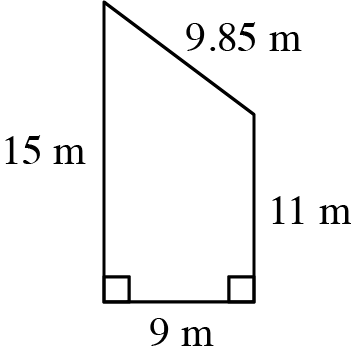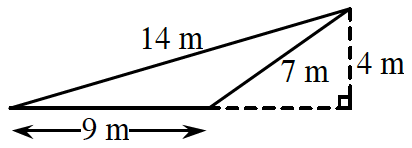### Home > CC1MN > Chapter 10 > Lesson 10.3.2 > Problem10-91

10-91.

Find the perimeter and area of each figure below.

1.The formula to find the area of a trapezoid is

$\frac{1}{2}\cdot (\text{base}_1+\text{base}_2)\cdot \text{(height)}$

Find the perimeter by finding the sum of the given side lengths.

1.The formula to find the area of a triangle is

$\frac{1}{2}\cdot (\text{base})\cdot \text{(height)}$

Do not be confused by the fact that the height is outside the triangle. The formula still applies.

$\text{Perimeter}=30$ m
$\text{Area}=18$ m2